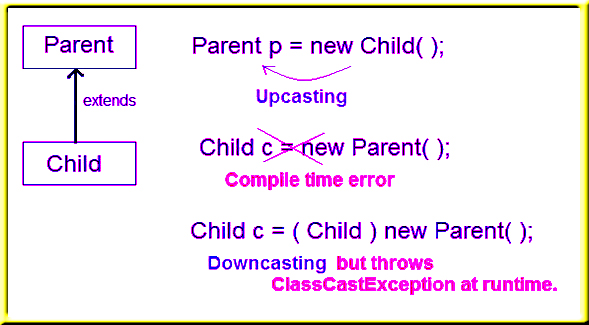# Java Tutorial

## Java instanceof

In Java, instanceof operator is used to check the type of an object at runtime. It is the means by which your program can obtain run-time type information about an object. instanceof operator is also important in case of casting object at runtime. instanceof operator return boolean value, if an object reference is of specified type then it return true otherwise false.

##### Example
```
public class Test
{
public static void main(String[] args)
{
Test t= new Test();
System.out.println(t instanceof Test);
}
}
```

##### Output:
```

true

```##### Example
```
class Parent{ }

public class Child extends Parent
{
public void check()
{
System.out.println("Sucessfull Casting");
}

public static void show(Parent p)
{
if(p instanceof Child)
{
Child b1=(Child)p;
b1.check();
}
}

public static void main(String[] args)
{

Parent p=new Child();

Child.show(p);

}
}
```

##### Output:
```

Sucessfull Casting

```
##### More example of instanceof operator
```
class Parent{}

class Child1 extends Parent{}

class Child2 extends Parent{}

class Test
{
public static void main(String[] args)
{
Parent p =new Parent();
Child1 c1 = new Child1();
Child2 c2 = new Child2();

System.out.println(c1 instanceof Parent);  //true
System.out.println(c2 instanceof Parent);  //true
System.out.println(p instanceof Child1);  //false
System.out.println(p instanceof Child2);  //false

p = c1;
System.out.println(p instanceof Child1);  //true
System.out.println(p instanceof Child2);  //false

p = c2;
System.out.println(p instanceof Child1);  //false
System.out.println(p instanceof Child2);  //true

}

}

```

```
true
true
false
false
true
false
false
true

```
##### Another example of java instanceof operator
```
class Animal{}
class Dog1 extends Animal{//Dog inherits Animal

public static void main(String args[]){
Dog1 d=new Dog1();
System.out.println(d instanceof Animal);//true
}
}
```

```

Output:true
```
##### instanceof in java with a variable that have null value

If we apply instanceof operator with a variable that have null value, it returns false. Let's see the example given below where we apply instanceof operator with the variable that have null value.

```
class Dog2{
public static void main(String args[]){
Dog2 d=null;
System.out.println(d instanceof Dog2);//false
}
}
```

```

false

```
##### Downcasting with java instanceof operator

When Subclass type refers to the object of Parent class, it is known as downcasting. If we perform it directly, compiler gives Compilation error. If you perform it by typecasting, ClassCastException is thrown at runtime. But if we use instanceof operator, downcasting is possible.

```
Dog d=new Animal();//Compilation error
```

If we perform downcasting by typecasting, ClassCastException is thrown at runtime.

```
Dog d=(Dog)new Animal();
//Compiles successfully but ClassCastException is thrown at runtime
```
##### Possibility of downcasting with instanceof
```
class Animal { }

class Dog3 extends Animal {
static void method(Animal a) {
if(a instanceof Dog3){
Dog3 d=(Dog3)a;//downcasting
System.out.println("ok downcasting performed");
}
}

public static void main (String [] args) {
Animal a=new Dog3();
Dog3.method(a);
}

}
```

##### Output:
```

ok downcasting performed
```
##### Downcasting without the use of java instanceof
```
class Animal { }
class Dog4 extends Animal {
static void method(Animal a) {
Dog4 d=(Dog4)a;//downcasting
System.out.println("ok downcasting performed");
}
public static void main (String [] args) {
Animal a=new Dog4();
Dog4.method(a);
}
}
```

##### Output:
```
ok downcasting performed
```

Let's take closer look at this, actual object that is referred by a, is an object of Dog class. So if we downcast it, it is fine. But what will happen if we write:

```
Animal a=new Animal();
Dog.method(a);
//Now ClassCastException but not in case of instanceof operator
```
##### Understanding Real use of instanceof in java
```
interface Printable{}
class A implements Printable{
public void a(){System.out.println("a method");}
}
class B implements Printable{
public void b(){System.out.println("b method");}
}

class Call{
void invoke(Printable p){//upcasting
if(p instanceof A){
A a=(A)p;//Downcasting
a.a();
}
if(p instanceof B){
B b=(B)p;//Downcasting
b.b();
}

}
}//end of Call class

class Test4{
public static void main(String args[]){
Printable p=new B();
Call c=new Call();
c.invoke(p);
}
}
```

##### Output:
```

b method
```